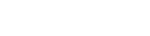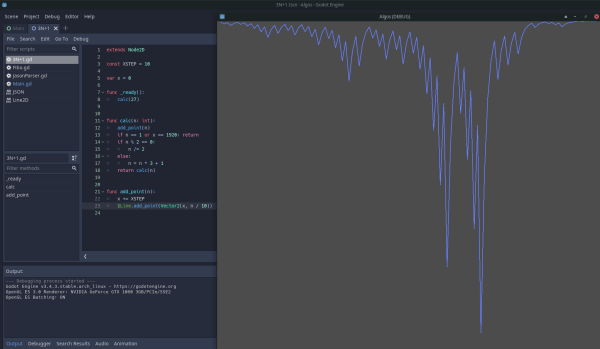# Godot Recursive Functions

A recursive function calls itself which can be useful to evaluate a series of calculations or traverse a tree-like data structure. Applications include solving puzzles or calculating a number from a mathematical series such as for estimating PI.

Each time the function is called, it will evaluate some code to determine if it needs to call the function again with new inputs, or return a value.

On each call to the function, the return point (address) is stored on the stack in memory. And, on return, the stack is unwound returning again and again to after the point at which the function was called. This continues until the original call to the function was made.

You can imagine that this could cause a stack overflow error, so we must have a condition to eventually return and complete the recursion. This condition may be tested at the point of entry to the function.

Now let’s look at some examples.

## Fibonacci Number Generator in Godot

A Fibonacci Number series is a sequence of numbers where the next number is the sum of the preceding 2 numbers starting with 0, 1.

``````func _ready():
print(fibo(16))

func fibo(n):
# If n is 0 or 1 return 1
if n < 2:
return 1
# Calculate the number
return fibo(n - 1) + fibo(n - 2)
``````

You can see here that the function is called twice by itself to recursively calculate the number.

This is a simple solution, but very inefficient since many repeated calculations are made. Calls are made with `n - 1` followed by calls with `n - 2`, but these also cause calls with `n - 1` which in turn cause calls with `n - 2` etc.

We may trace the progress by tagging the calls with side `a` and side `b` markers to make sense of what is going on.

``````func _ready():
print(fibo(5))

func fibo(n, from = ""):
prints(n, from)
if n < 2:
return 1
return fibo(n - 1, "a") + fibo(n - 2, "b")
``````

This will print a list of input numbers and which side they came from. You will be able to observe many repeated calculations.

With large numbers, this solution will magnify an already long time to complete the calculations. So this demonstrates a problem to look out for when implementing recursion.

## Improved Fibonacci Number Calculator

We may store newly calculated numbers in an array. Then there is no need to call the function if a number was previously calculated. This will result in much faster computation.

``````func _ready():
print(fibo(34))

func fibo(n: int, arr := [1, 1]):
if arr.size() <= n: # This is a new number
n = fibo(n - 1, arr) + fibo(n - 2, arr)
arr.push_back(n)
else:
n = arr[n] # Access a previously calculated number
return n
``````

Arrays are passed by reference, so the array values will be preserved throughout the process of recursively calling the function.

Also, here I have added type hinting to the input parameters to help with the editor (IDE) assisting us with code entry and error checking.

Furthermore, the initial state of the array values have been set inside of the function so as to allow calling the function as expected with only the input number.

## Calculator for the value of Pi

This script demonstrates evaluating a mathematical series to approximate the value of Pi.

``````const MAX_N = 1000

var pi = calc_pi(2, 3.0, +1.0, 2.0)
print(pi)

func calc_pi(n, pi, sign_, d):
if n > MAX_N:
return pi
else:
return calc_pi(n + 1, pi + 4.0 * sign_ / (d * (d + 1.0) * (d + 2.0)), -sign_, d + 2.0)
``````

The sign value is toggled between plus and minus on each iteration. The recursion is terminated when a particular number of iterations have been made to avoid a stack overflow and this also affects the accuracy of the approximation.

The trailing dash is added to avoid a clash with a name of an existing Godot function.

## Collatz conjecture 3n + 1 code

This script demonstrates a simple recursive algorithm to calculate numbers in a series where odd numbers produce `3n + 1` and even numbers are divided by 2. It is observed that any positive starting integer eventually ends up at 1. But (at the time of writing) nobody has been able to prove this despite great efforts to do so.``````extends Node2D

# Has a Line2D child node to plot a curve using each number of the series that tends to 1

const XSTEP = 10

var x = 0

calc(211)

func calc(n: int):
if n == 1 or x == 1920: return
# Divide even numbers by 2
if n % 2 == 0:
n /= 2
else:
n = n * 3 + 1
return calc(n)